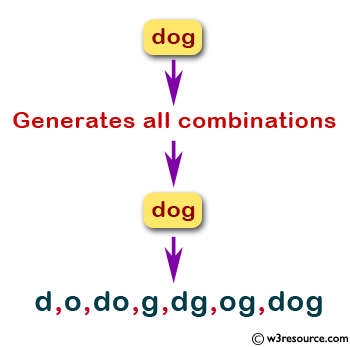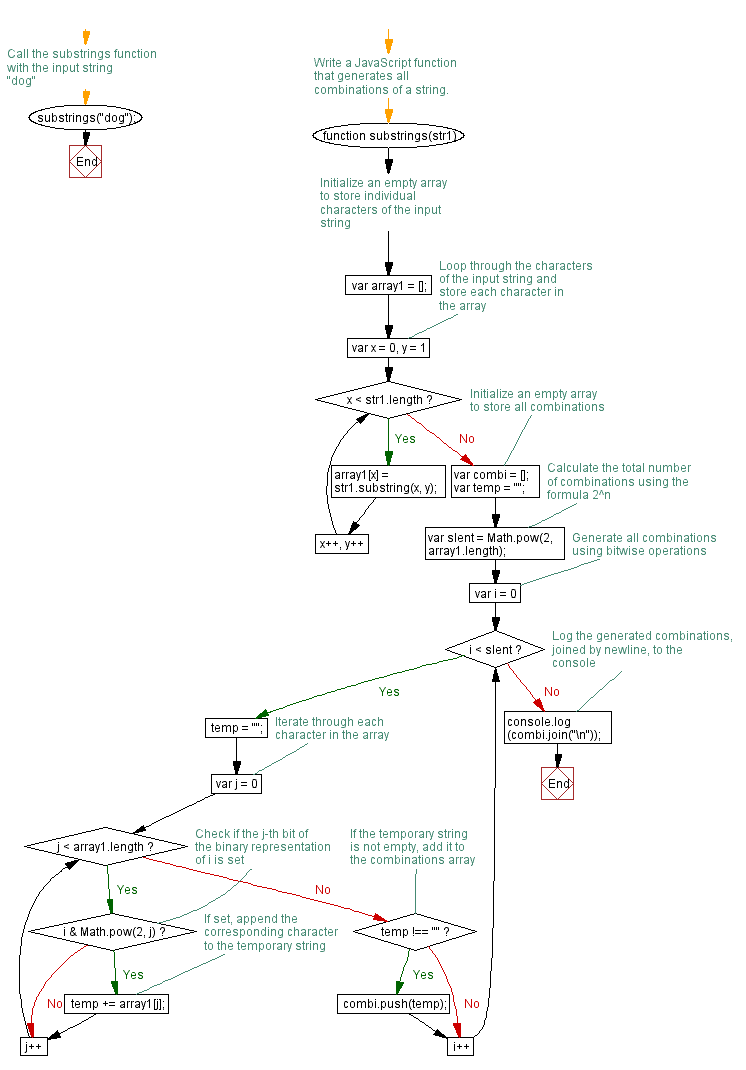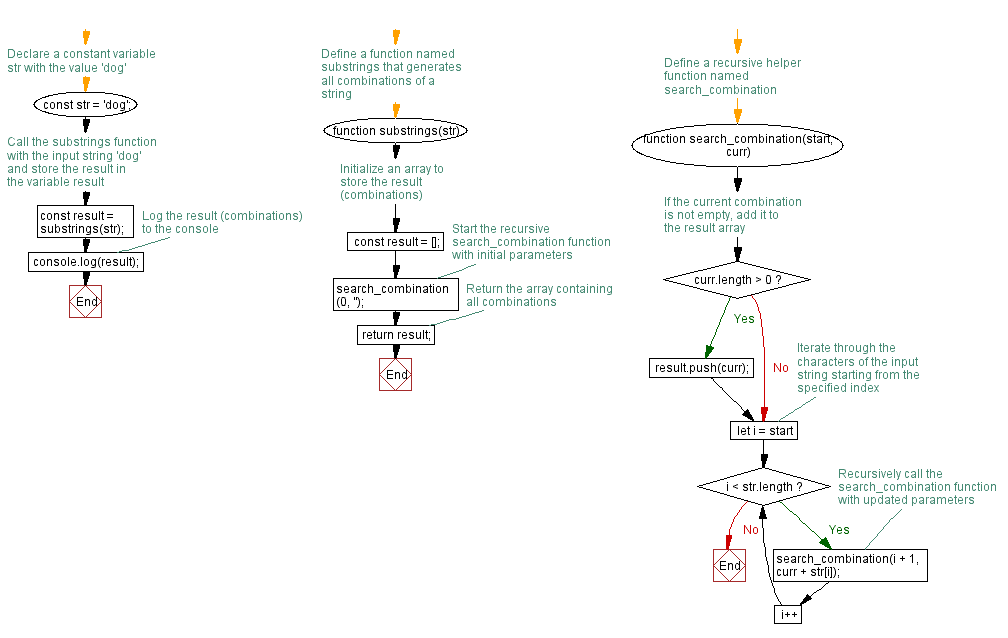# JavaScript: Generates all combinations of a string

## JavaScript Function: Exercise-3 with Solution

Write a JavaScript function that generates all combinations of a string.
Example string: 'dog'
Expected Output: d,o,do,g,dg,og,dog

Pictorial Presentation:Sample Solution-1:

JavaScript Code:

``````//Write a JavaScript function that generates all combinations of a string.
function substrings(str1)
{
var array1 = [];
for (var x = 0, y=1; x < str1.length; x++,y++)
{
array1[x]=str1.substring(x, y);
}
var combi = [];
var temp= "";
var slent = Math.pow(2, array1.length);

for (var i = 0; i < slent ; i++)
{
temp= "";
for (var j=0;j<array1.length;j++) {
if ((i & Math.pow(2,j))){
temp += array1[j];
}
}
if (temp !== "")
{
combi.push(temp);
}
}
console.log(combi.join("\n"));
}

substrings("dog");
```
```

Sample Output:

```d
o
do
g
dg
og
dog
```

Flowchart:Live Demo:

See the Pen JavaScript -Check whether a passed string is palindrome or not-function-ex- 2 by w3resource (@w3resource) on CodePen.

Improve this sample solution and post your code through Disqus

Sample Solution-2:

JavaScript Code:

``````function substrings(str) {
const result = [];

function search_combination(start, curr) {
if (curr.length > 0) {
result.push(curr);
}
for (let i = start; i < str.length; i++) {
search_combination(i + 1, curr + str[i]);
}
}
search_combination(0, '');
return result;
}
const str = 'dog';
const result = substrings(str);
console.log(result);
```
```

Sample Output:

```["d","do","dog","dg","o","og","g"]
```

Explanation:
The above substrings() function takes a string as input and uses a function called search_combination () to recursively generate the combinations. The start parameter keeps track of the index in the input string where the function should start adding characters to the current combination, and the curr parameter stores the current combination being generated.

At each step, the function checks if the current combination is not empty and adds it to the result array if it is not. Then, the function loops over the remaining characters in the input string starting from the current index start, and recursively calls search_combination() function with the updated start and curr parameters. This generates all possible combinations of the characters in the string.

Flowchart:Live Demo:

See the Pen javascript-function-exercise-3-1 by w3resource (@w3resource) on CodePen.

Improve this sample solution and post your code through Disqus

What is the difficulty level of this exercise?

Test your Programming skills with w3resource's quiz.

﻿